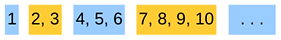Positive Integers

The Last NumberOn a piece of paper write all the integers from 1 to 111. Cross out any two of them and replace them with their sum. Repeat until you have only one number left.

What is the last number?

A Barren Number Line

Slide positive integers off the number line, and place them into boxes. The first number goes into the first box, the next two numbers go into the second box, etc.What is the smallest number that goes into the 99th box?

A player has to write on the board the sum of any two numbers that are already on the board.A round starts with numbers 7 and 17. Which of the following numbers can not be on the board at any time during this round: 119, 123, 188, or 205?

Partitioning Integers

All positive integers are separated into a blue bin and a purple bin according to the pattern below.a.) Which bin contains the number 22?

b.) Is number 22 in the same bin with 2020?

Last DigitWhich of the following choices is correct?

a.) The last digit of the sum is 1.

b.) The last digit of the sum is 2.

c.) The last digit of the sum is 3.

d.) The last digit of the sum is 4.